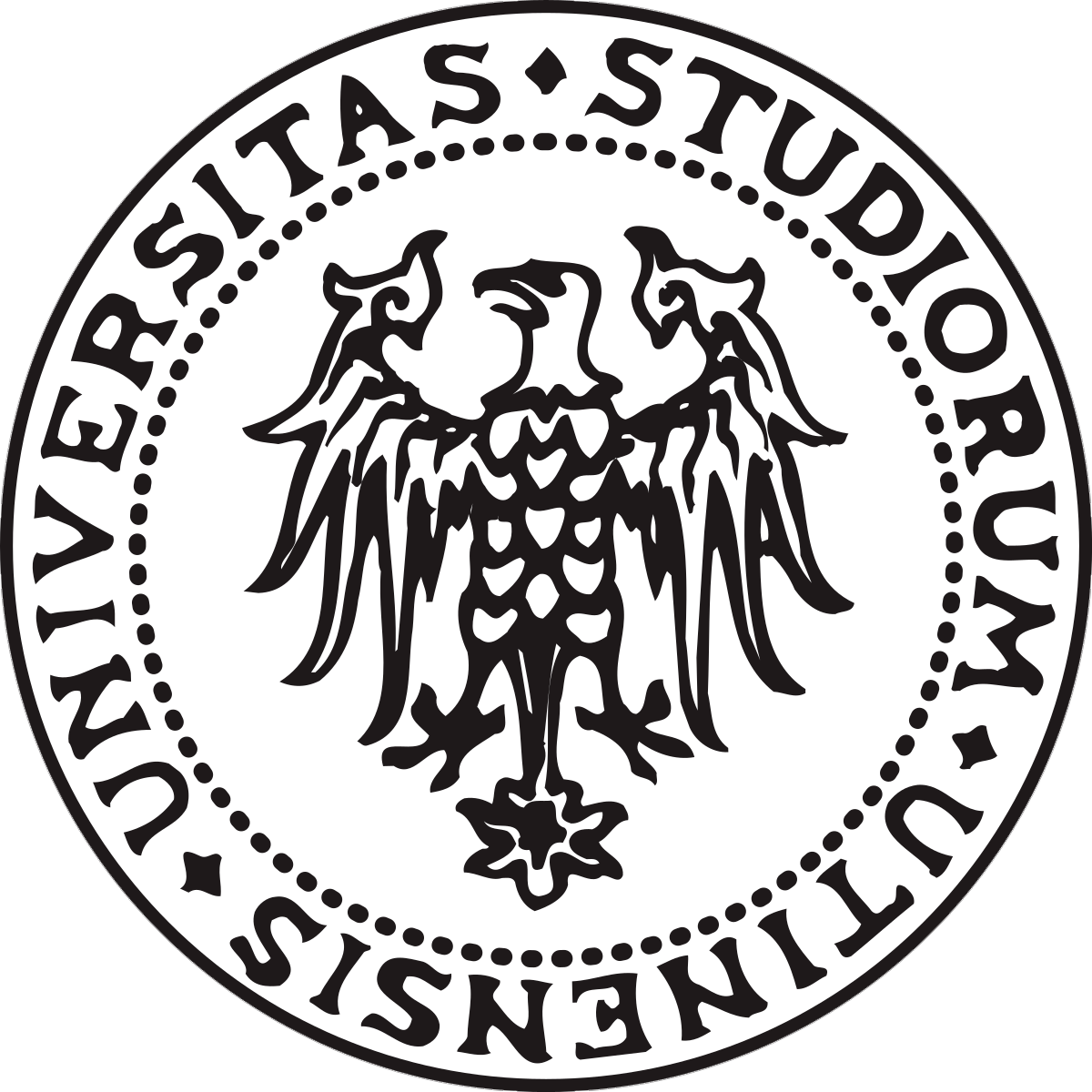## Project

### The idea

For the execution of the project it was chosen to use two Arduino boards connected to each other via the radio protocol. An Arduino board is placed on the existing drone and has a GPS module and radio antenna mounted. The second card is connected to the PC and has the radio receiver module. The PC then reprocesses the information received through a Python script and shows on the screen the position of the drone and the probability that the mine is at that point. For this project, the mine presence information is simulated by generating a random number between 0 and 1 via the Arduino board. The number describes the probability of the presence of the mine.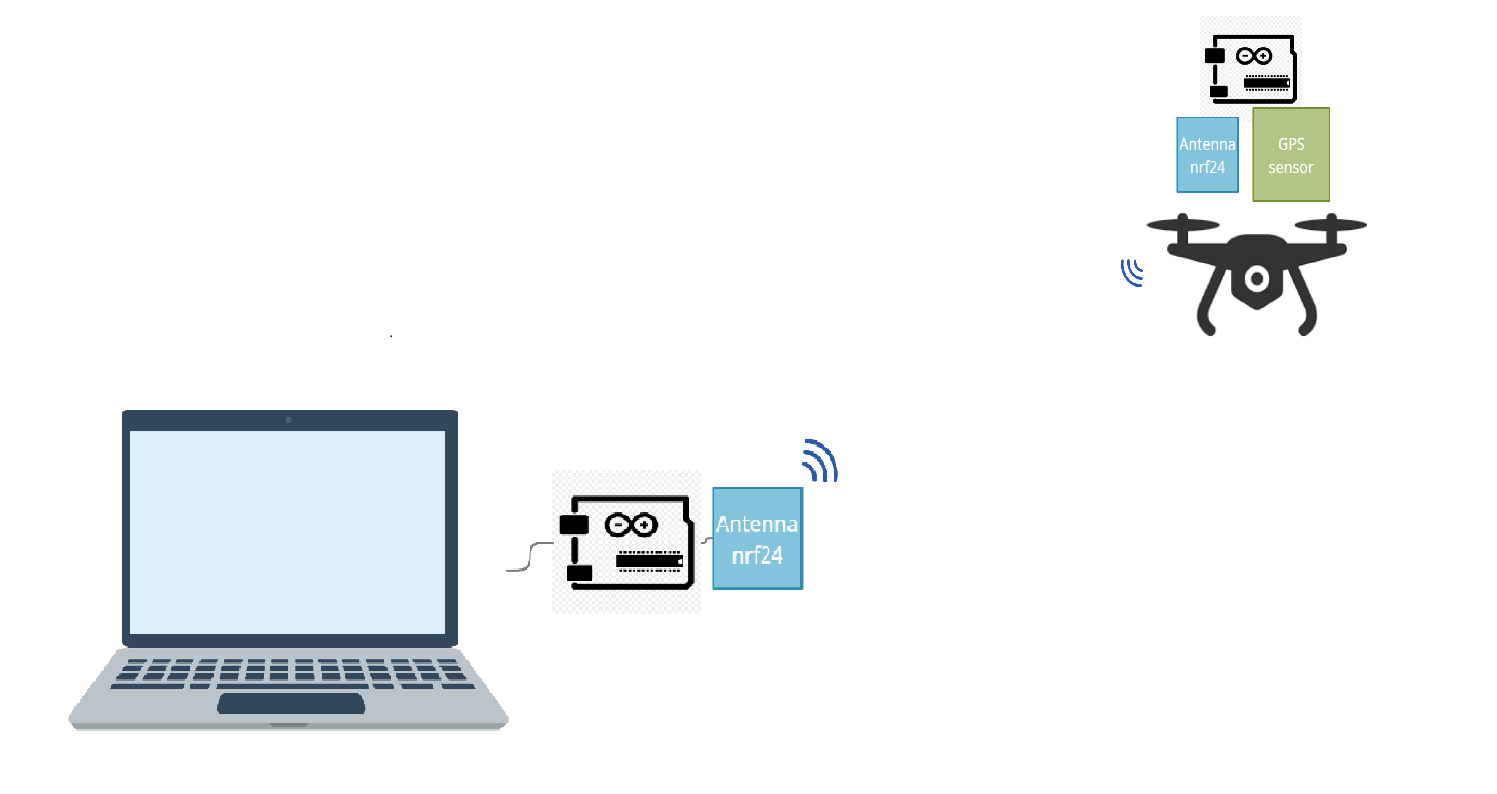The following sections show the hardware diagrams created, the Arduino code and the Python code.

### Hardware scheme

The components are:

• Arduino nano
•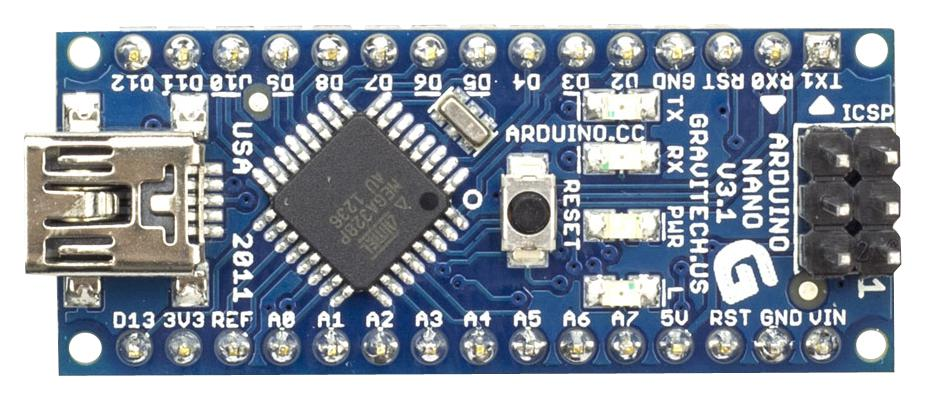The Arduino Nano is Arduino's classic breadboard friendly designed board with the smallest dimensions. The Arduino Nano comes with pin headers that allow for an easy attachment onto a breadboard and features a Mini-B USB connector. The ATMega328 CPU runs with 16 MHz and features 32 KB of Flash Memory (of which 2 KB used by bootloader). At this link you can see all the specifications: https://docs.arduino.cc/hardware/nano

• Arduino UNO
•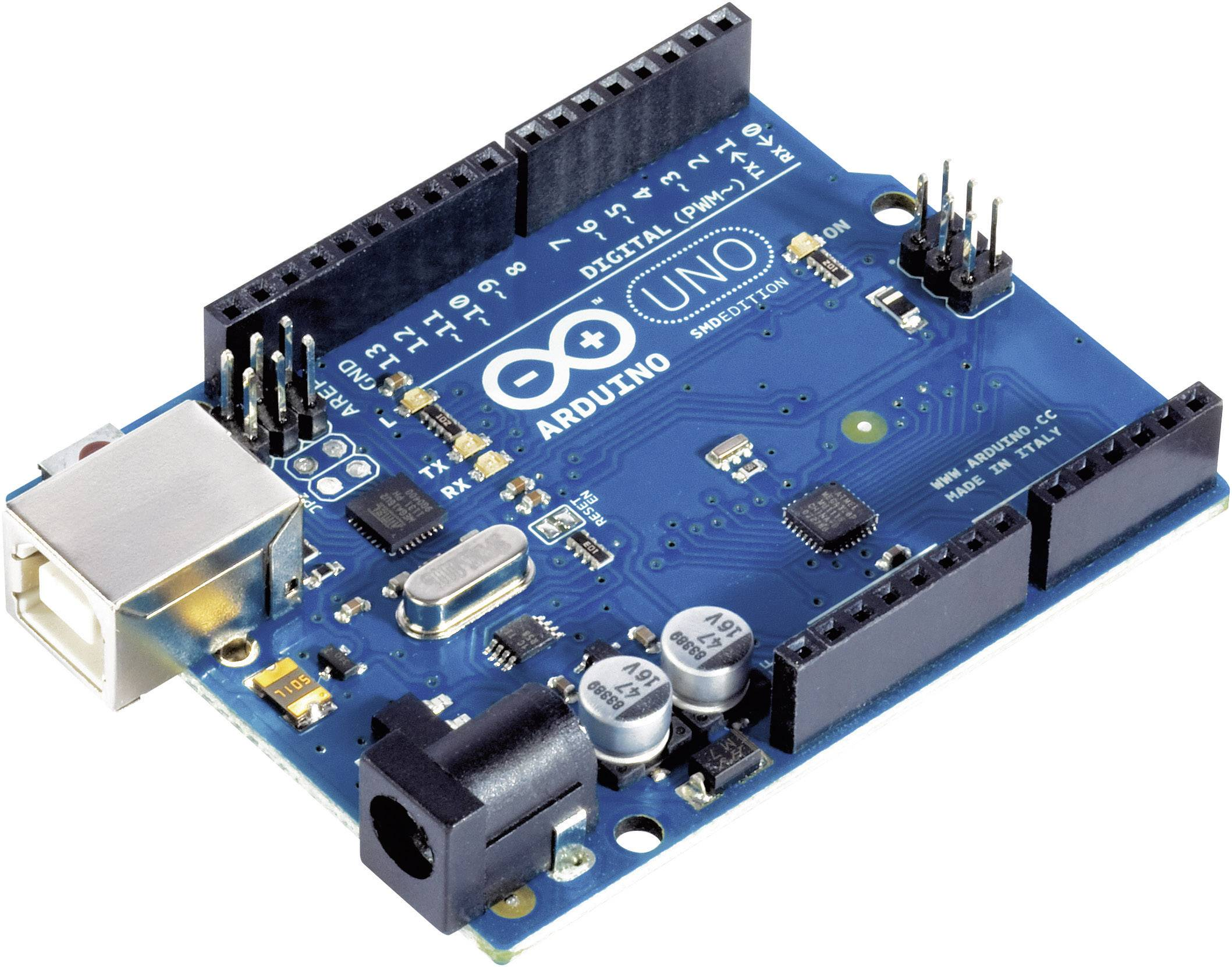Arduino UNO is a microcontroller board based on the ATmega328P. It has 14 digital input/output pins (of which 6 can be used as PWM outputs), 6 analog inputs, a 16 MHz ceramic resonator, a USB connection, a power jack, an ICSP header and a reset button.
The Arduino UNO is a board to get started with electronics and coding. If this is your first experience tinkering with the platform, the UNO is the most robust board you can start playing with. The UNO is the most used and documented board of the whole Arduino family.
At this link you can see all the specifications: https://docs.arduino.cc/hardware/uno-rev3

• 2 antenne nrf24
•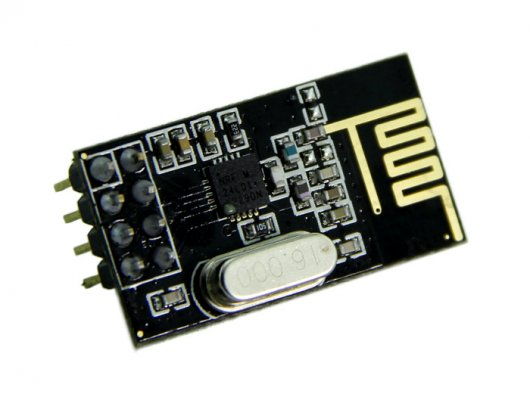NRF24L01 works in 2.4-2.5GHz ISM BAND global wireless single-chip transceiver. This is a cheap and easy to use RF wireless transceiver module based on the popular nRF24L01 from Nordic Semiconductor. It works at the 2.4 GHz frequency range and has a high on air data rate of up to 1 - 2 Mbps and comes with an onboard antenna. It's bidirectional. It has a standard SPI interface, which means you can directly interface this module to any microcontroller without the need for any external circuit.
• 2 100µF capacitors
•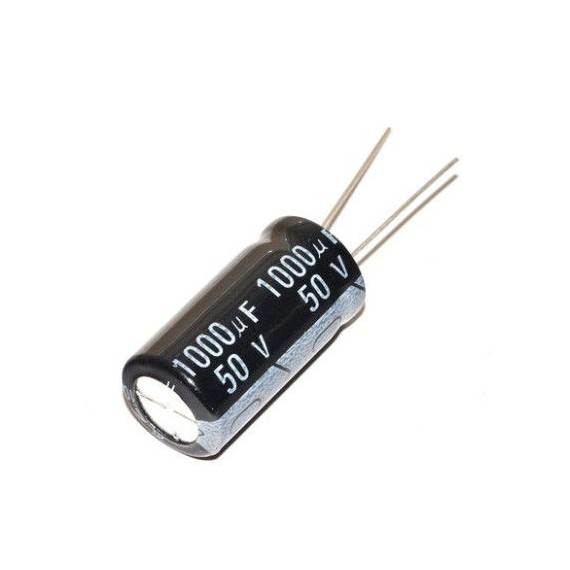• a sensor BN-220 GPS
•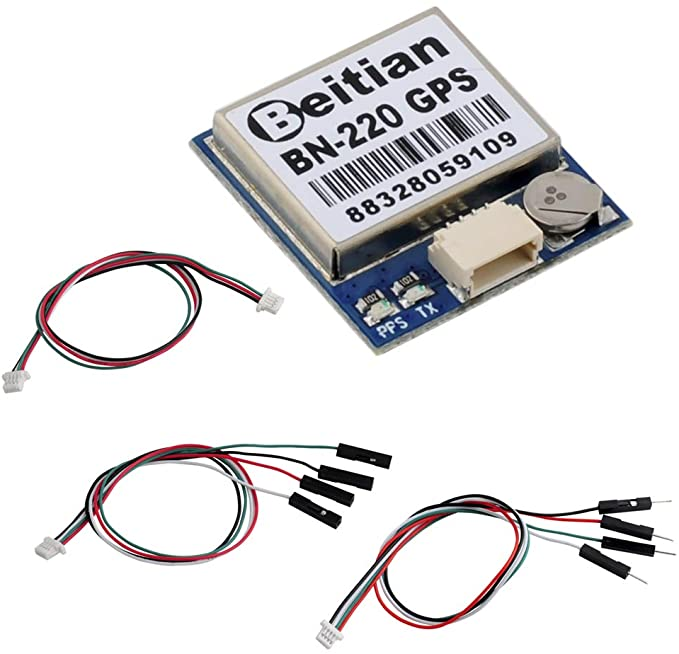It's a GPS receiver module complete with connection cable for FC APM and suitable for installation and use on Drones

• 2 9V batteries
•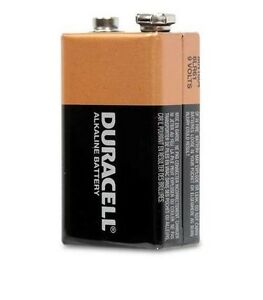• 2 buck convertitor DC-DC LM2596
•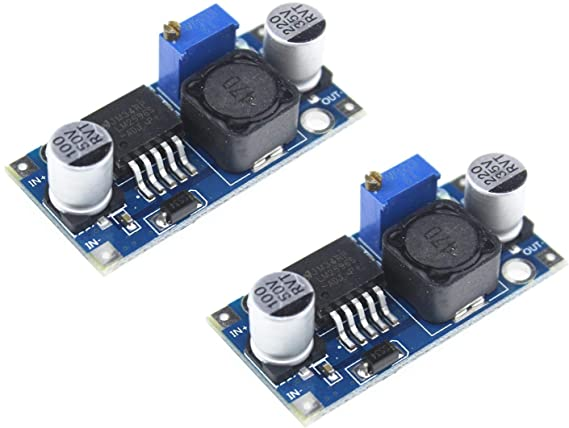The LM2596 Adjustable DC-DC Step Down converter allows you to step down (Buck) an input voltage range of 3 - 40V to an output voltage range of 1.23 - 37V up to a maximum of 3 Amps. The module includes Under voltage protection, current limiting and thermal overload protection. To adjust the output voltage, turn the onboard trimpot.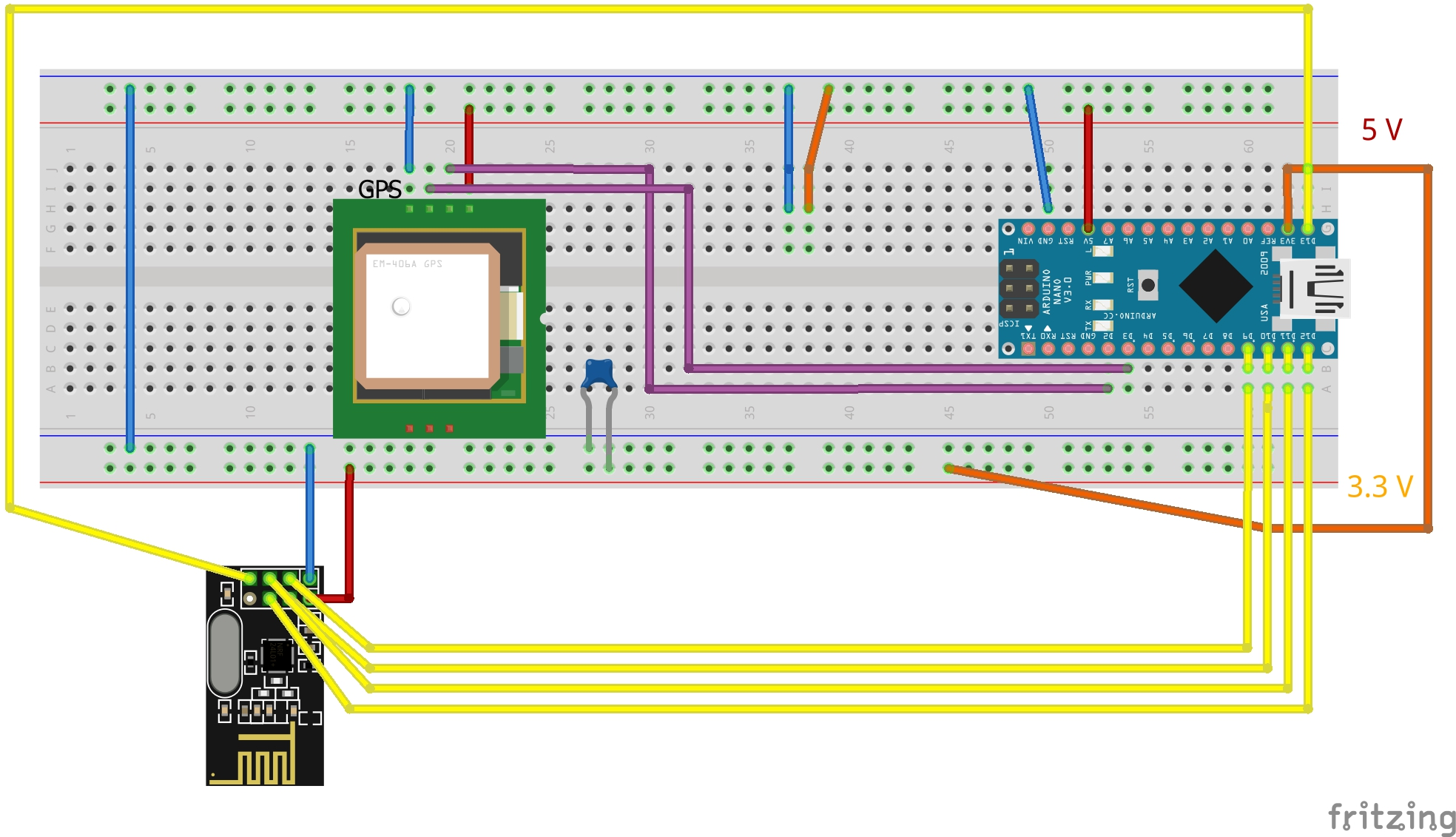Schematic of the modulo on the Arduino Board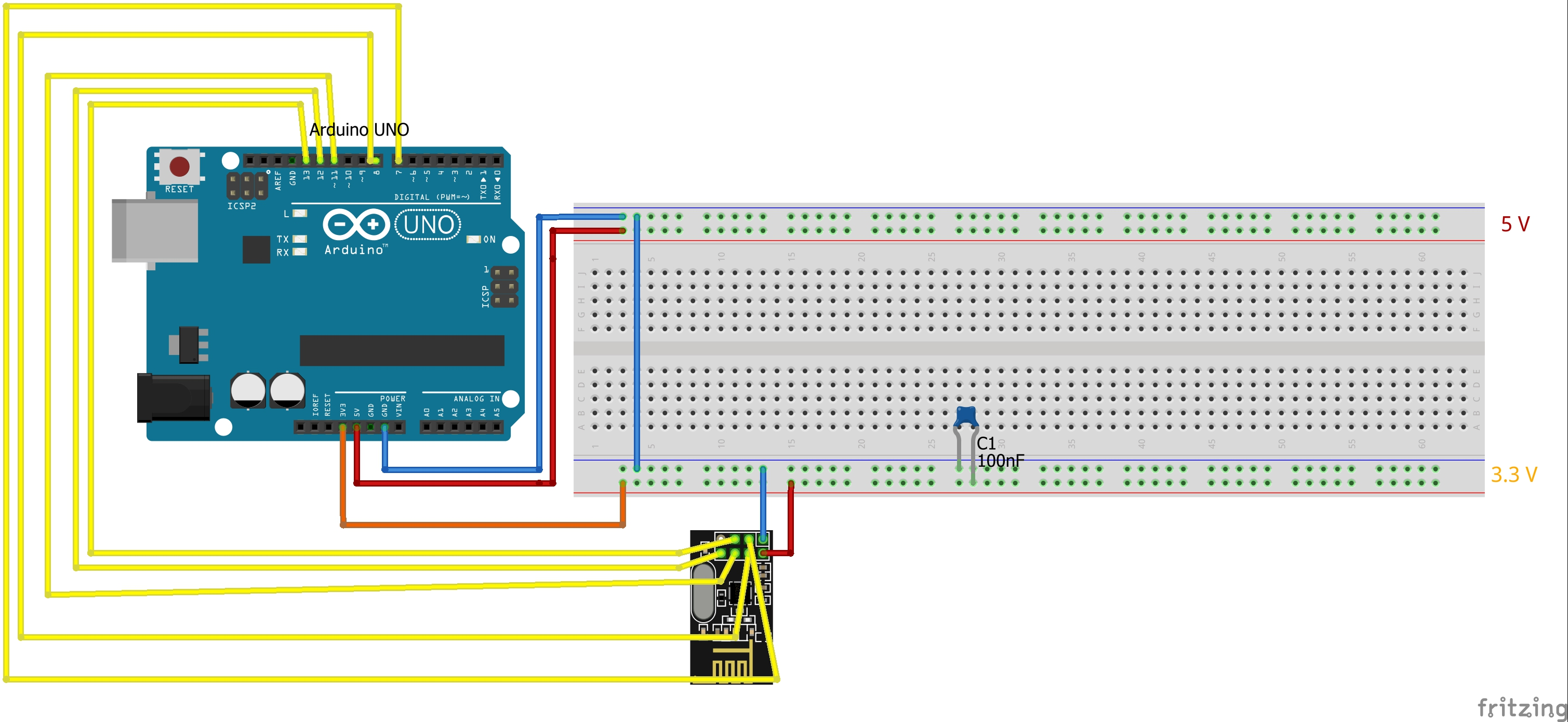Schematic of the modulo on the floor and connected to PC

### Python code

The code implemented on Python must:

• Read strings of data from the serial port of Arduino Uno connected to USB port
• Extract latitude, longitude and value of the samples taken from the board of drone
• Create the background of the map and move the drone image in real-time
• Set points inside the map based on the probability of finding a mine underground
• Save the results of the acquisition inside a file
Initially, we have to know in which port of our operating system is connected the board of Arduino. This is made automatically by the following code (in case of multiple Arduino connected to the PC we use the first):

``````
import warnings
import serial
import serial.tools.list_ports

arduino_ports = [
p.device
for p in serial.tools.list_ports.comports()
if 'Arduino' in p.description
]
if not arduino_ports:
raise IOError("No Arduino found")
if len(arduino_ports) > 1:
warnings.warn("Multiple Arduinos found - using the first")
``````

Now we know in which port we receive the data from Arduino. We have to create an instance of the serial port and read the lines arriving from the Arduino connected on the drone.

``````
ser = serial.Serial(arduino_ports)
print("connected to Arduino on: " + ser.portstr)
cc = line[2:][:-6]
``````

Inside the cc variable we have a string with this format: `3,46.081236,13.212089,0.211`
Where the first element is the time, the second element is the latitude, the third element is longitude and the latter is the value.
The creation of the map is made by Dash-Leaflet, a wrapper of Leaflet that is an open-sorce library written in JavaScript used for the generation of interactive maps. The main components of the map is the app instance and the app layout that contains the HTML, Dash and Dash-Leaflet components of the web server application. The components of the real-time map application are the following:

``````
# Create the app.
app = Dash(external_scripts=[chroma], prevent_initial_callbacks=True)
app.layout = html.Div([
html.Button(id="button", children="Start/Pause", className="button"),
dcc.Interval(
id='interval-component',
interval=1000, # in milliseconds
n_intervals=0,
disabled=True
),
dcc.Interval(
id='interval-component2',
interval=1000, # in milliseconds
n_intervals=0
),
html.Br(),
html.Hr(),
dl.Map([dl.ImageOverlay(url=image_url, bounds=image_bounds, id="drone", zIndex=1000), dl.TileLayer(), geojson, colorbar],
bounds=image_bounds, id="map",
style={'width': '100%', 'height': '80vh', 'margin': "auto", "display": "block"}
)
], style={'text-align': 'center'})

if __name__ == '__main__':
app.run_server(port = 8050, dev_tools_ui=True,
``````

In the first line we set an instance of the Dash-Leaflet application, in the second line we create these components: a button that can start and pause the acquisition of the probability values coming from the drone, one interval component that moves the drone image based on its position, one interval component that starts counting every second when the button is pressed and the map component that contains the map background, the drone image and the sampled points. The last line starts a flask server in local mode in the domain http://127.0.0.1:8050. The result should look like this: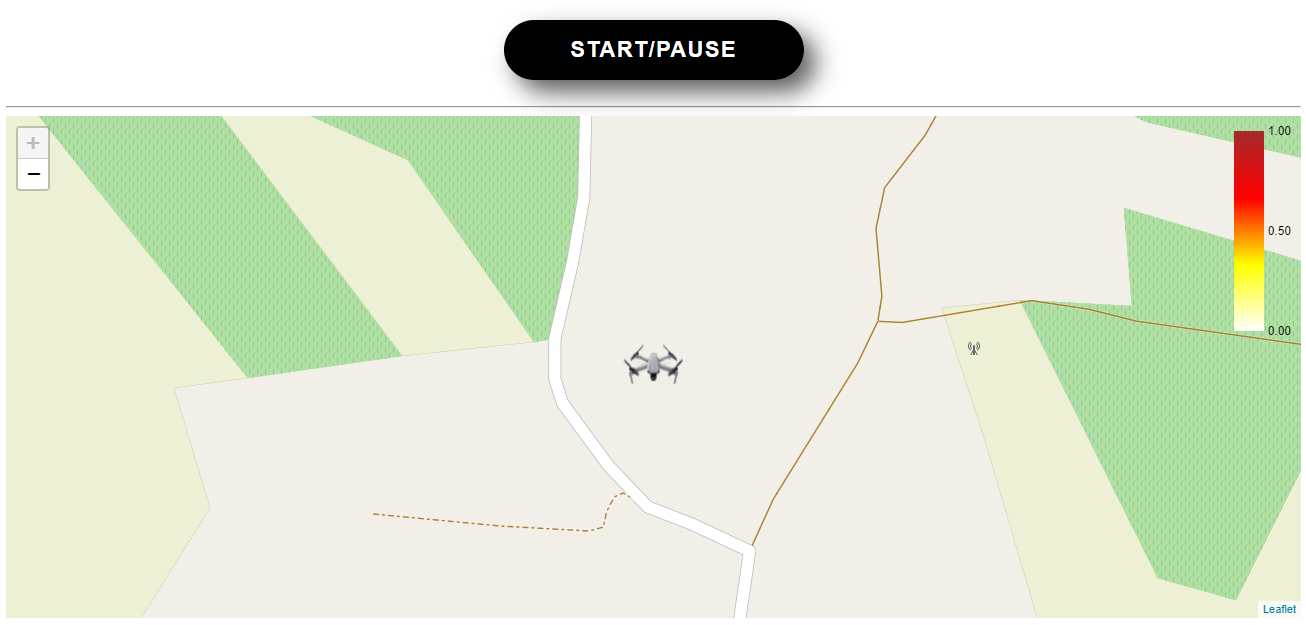The sample points indicating the probability of finding a mine in a certain location are drawn using GeoJSON objects, this is a format for encoding a variety of geographic data structures based on the JSON format. In particular, using this application, we want to draw circles for each sampled position showing the time, the position and the value of the sample. If the sample are stored inside a Pandas DataFrame named `df` with columns time, latitude, longitude and value, the markers are drawn inside the map with the following code:

``````
dicts = df.to_dict('records')
for item in dicts:
item["tooltip"] = f"time={item['time']}, latitude={item['latitude']}, longitude={item['longitude']}, probability={item['probability']}"
geojson = dlx.dicts_to_geojson(dicts, lat="latitude", lon="longitude")  # convert to geojson
geobuf = dlx.geojson_to_geobuf(geojson)  # convert to geobuf

# Geojson rendering logic, must be JavaScript as it is executed in clientside.
point_to_layer = assign("""function(feature, latlng, context){
const {min, max, colorscale, circleOptions, colorProp} = context.props.hideout;
const csc = chroma.scale(colorscale).domain([min, max]);  // chroma lib to construct colorscale
circleOptions.fillColor = csc(feature.properties[colorProp]);  // set color based on color prop.
var circle = L.circleMarker(latlng, circleOptions);
return circle.bringToBack()  // sender a simple circle marker.
}""")
# Create geojson.
geojson = dl.GeoJSON(data=geobuf, id="geojson", format="geobuf",
zoomToBounds=True,  # when true, zooms to bounds when data changes
options=dict(pointToLayer=point_to_layer),  # how to draw points
min=0, max=1, colorscale=colorscale)
)
``````

In order to update the map in real time using the data arriving from the serial port of Arduino, some callback functions of the app object have been developed. Callback functions of a Dash app are functions that are automatically called by Dash whenever an input component's property changes, in order to update some property in another component (the output). Input and Outputs of a callback functions are the Dash HTML components modules by means of their attributes like style, className and id. The Dash Core Components (dash.dcc) module generates higher-level components like controls and graphs. A callback function is normally used as a decorator. In the following it is shown the part of real-time updating.

#### Start/Pause button

The button above the map is used for starting and stopping the live plotting of the points on the map. This is made by counting how many times the button has been pressed and controlling the interval-component that is responsible for the points. Furthermore, when we set the system in pause, the DataFrame of the sampled points is saved in a local file.

``````
@app.callback(
Output('interval-component', 'disabled'),
Input('button', 'n_clicks')
)
def play_pause(n_clicks):
if n_clicks % 2 == 0:
return n_clicks % 2 == 0
``````

#### Change the position of the drone image

In this part, the code read every second through the interval-component2 the string arriving from the serial port. This function can move the drone image in the last position captured by the GPS sensor and center the map in that point.

``````
@app.callback(
Output('drone', 'bounds'),
Output('map', 'bounds'),
[Input('interval-component2', "n_intervals")]
)
def move_drone(n_intervals):
global df
global punto_corrente

if line[:2] == "b\'":
cc = line[2:][:-6]
punto_corrente = cc.split(",")
punto_corrente = [float(element) for element in punto_corrente]
punto_corrente = round(punto_corrente, 5)

df = df.append(pd.DataFrame([punto_corrente], columns=df.columns), ignore_index=True)
image_bounds = [[df.iloc[len(df)-1].latitude - dd, df.iloc[len(df)-1].longitude - dd], [df.iloc[len(df)-1].latitude + dd, df.iloc[len(df)-1].longitude + dd]]

return(image_bounds, image_bounds)
``````

#### Draw the sampled points

This is the most important part of the Python code. This section is responsible for drawing the points on the background map where the drone image is located. The idea is that one point is considered accettable if the drone is inside a circular region with radius set by the constant raggio_cerchio for at least 5 points (i.e. 5 seconds). In this way, the measure of the mine sensor is more accurate, therefore we can be sure that someone is able to know the probability of the presence of a mine ain a certain position. This can be done by appending to the list lista_coordinate the coordinates and the value for every acquisition. If the distance between the previous point and the new point is greater than raggio_cerchio, the new point is taken as a reference for next acquisitions. After the acquisition of 5 points inside the same circle, if there isn't a point already existing it will be created, otherwise an average will be made with the previous samples. The constant raggio_cerchio is set by default with the value of 3 meters. The distances from two points are computed by the function `conversione_latlong_metri` that takes as arguments the two DataFrame with the positions in latitude and longitude and returns the distance in meters. Once the new points are computed, the function returns the GeoJSON object with the data updated.

``````
@app.callback(
Output('geojson', 'data'),
[Input('interval-component', 'n_intervals')]
)
def calcolo_punti(value):
global df
global punto_corrente_ok
global lista_coordinate
global cc_df

punto_precedente = punto_corrente
df_punto_corrente = pd.DataFrame([punto_corrente], columns=cols)
if conversione_latlong_metri(df_punto_corrente, punto_precedente) <= raggio_cerchio:
lista_coordinate = lista_coordinate.append(df_punto_corrente, ignore_index=True)
else:
lista_coordinate = df_punto_corrente

if (len(lista_coordinate) >= 5):
punto_corrente_ok = [lista_coordinate.loc[0, 'time'], lista_coordinate.loc[0, 'latitude'], lista_coordinate.loc[0, 'longitude'], lista_coordinate['probability'].mean()]
lista_coordinate = pd.DataFrame(columns=cols)
distanza_metri = conversione_latlong_metri(cc_df, punto_corrente_ok)
if np.all(distanza_metri >= 2 * raggio_cerchio):    # crea un nuovo punto sulla mappa
cc_df = cc_df.append(pd.DataFrame([punto_corrente_ok + [0, 0]], columns=cc_df.columns), ignore_index=True)
elif np.any(distanza_metri < raggio_cerchio):       # aggiorna il valore di probabilità del punto già esistente
index_min = np.argmin(np.sqrt(np.power((cc_df.latitude - punto_corrente_ok), 2) + np.power((cc_df.longitude - punto_corrente_ok), 2)))
cc_df.loc[index_min, 'cumsum'] = cc_df.loc[index_min, 'cumsum'] + punto_corrente_ok
cc_df.loc[index_min, 'n_meas'] = cc_df.loc[index_min, 'n_meas'] + 1
cc_df.loc[index_min, 'probability'] = cc_df.loc[index_min, 'cumsum'] / cc_df.loc[index_min, 'n_meas']

dicts = cc_df.to_dict('records')
for item in dicts:
item["tooltip"] = f"time={item['time']}, latitude={item['latitude']}, longitude={item['longitude']}, probability={item['probability']}"
geojson = dlx.dicts_to_geojson(dicts, lat="latitude", lon="longitude")
geobuf = dlx.geojson_to_geobuf(geojson)  # convert to geobuf

return(geobuf)
``````

### Arduino code

The script for the board on drone is:

``````
#include <Wire.h>
#include <SPI.h>
#include <nRF24L01.h>
#include <RF24.h>
#include <TimeLib.h>
#include <printf.h>
#include <TinyGPS++.h>
#include <SoftwareSerial.h>

static const int RXPin = 2, TXPin = 3;
static const uint32_t GPSBaud = 9600;

TinyGPSPlus gps;

SoftwareSerial ss(RXPin, TXPin);

double valore_mina;
double latitudine;
double longitudine;
unsigned char b[sizeof(double)];
RF24 radio(7, 8); // CE, CSN

double myArray;
char secondo;
char terzo;
char quarto;

uint32_t timer;
double timer_reset, timer_testa;
uint8_t i2cData; // Buffer for I2C data

void setup() {
Serial.begin(115200);
ss.begin(GPSBaud);

timer = micros();
timer_reset = 0;
timer_testa = 0;
}

void loop() {

smartDelay(1000);
valore_mina = random(1000)/double(1000);

myArray = now();
myArray = gps.location.lat();
myArray = gps.location.lng();
myArray = valore_mina;

printf_begin();

for(int i=0; i<4; i++){
Serial.print(myArray[i]);
Serial.print(',');
}
Serial.println();
}

static void smartDelay(unsigned long ms)
{
unsigned long start = millis();
do
{
while (ss.available())
} while (millis() - start < ms);
}
``````

The script for the board connect to PC is :

``````
#include <SPI.h>
#include <printf.h>
#include <nRF24L01.h>
#include <RF24.h>
RF24 radio(9, 10); // CE, CSN

double remoteArray;
int bt;
void setup() {
Serial.begin(9600);
// Serial.println("Pronto..");
}

void loop() {
// Serial.println("Available");
printFloat(remoteArray, 11, 6); Serial.print(",");
printFloat(remoteArray, 11, 6); Serial.print(",");
printFloat(remoteArray, 11, 6); Serial.print(",");
printFloat(remoteArray, 11, 6);
Serial.println();
}

//printf_begin();

delay(1000);

}

static void printFloat(float val,int len, int prec)
{
Serial.print(val, prec);
int vi = abs((int)val);
int flen = prec + (val < 0.0 ? 2 : 1); // . and -
flen += vi >= 1000 ? 4 : vi >= 100 ? 3 : vi >= 10 ? 2 : 1;
}
``````

Polytechnic department of engineering and architecture

Università degli Studi di Udine